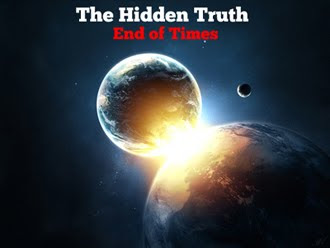# The Hidden Truth End of TimeThe Hidden Truth End of Time Part 1 of 39

The Hidden Truth End of Time Part 2 of 39

The Hidden Truth End of Time Part 3 of 39

The Hidden Truth End of Time Part 4 of 39

The Hidden Truth End of Time Part 5 of 39

The Hidden Truth End of Time Part 6 of 39

The Hidden Truth End of Time Part 7 of 39

The Hidden Truth End of Time Part 8 of 39

The Hidden Truth End of Time Part 9 of 39

The Hidden Truth End of Time Part 10 of 39

The Hidden Truth End of Time Part 11 of 39

The Hidden Truth End of Time Part 12 of 39

The Hidden Truth End of Time Part 13 of 39

The Hidden Truth End of Time Part 14 of 39

The Hidden Truth End of Time Part 15 of 39

The Hidden Truth End of Time Part 16 of 39

The Hidden Truth End of Time Part 17 of 39

The Hidden Truth End of Time Part 18 of 39

The Hidden Truth End of Time Part 19 of 39

The Hidden Truth End of Time Part 20 of 39

The Hidden Truth End of Time Part 21 of 39

The Hidden Truth End of Time Part 22 of 39

The Hidden Truth End of Time Part 23 of 39

The Hidden Truth End of Time Part 24 of 39

The Hidden Truth End of Time Part 25 of 39

The Hidden Truth End of Time Part 26 of 39

The Hidden Truth End of Time Part 27 of 39

The Hidden Truth End of Time Part 28 of 39

The Hidden Truth End of Time Part 29 of 39

The Hidden Truth End of Time Part 30 of 39

The Hidden Truth End of Time Part 31 of 39

The Hidden Truth End of Time Part 32 of 39

The Hidden Truth End of Time Part 33 of 39

The Hidden Truth End of Time Part 34 of 39

The Hidden Truth End of Time Part 35 of 39

The Hidden Truth End of Time Part 36 of 39

The Hidden Truth End of Time Part 37 of 39

The Hidden Truth End of Time Part 38 of 39

The Hidden Truth End of Time Part 39 of 39